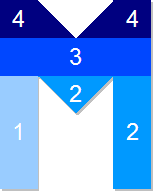#### You may also likeFour vehicles travelled on a road. What can you deduce from the times that they met?### Parabolic Patterns

The illustration shows the graphs of fifteen functions. Two of them have equations y=x^2 and y=-(x-4)^2. Find the equations of all the other graphs.### More Parabolic Patterns

The illustration shows the graphs of twelve functions. Three of them have equations y=x^2, x=y^2 and x=-y^2+2. Find the equations of all the other graphs.

# Mathsjam Jars

##### Age 14 to 16 Challenge Level:
Well done to Anurag and Christina for their solutions to this problem:

The seven letters that take the same time to fill up are: I,L,O,E,M,T and H, all with a volume $14$cm$^3$ and thus taking $14$ minutes to fill.

The letter S takes the longest to fill up ($14.5$cm$^3$, $14.5$ minutes to fill).
The letter V fills up first ($13$cm$^3$, $13$ minutes to fill).
The letter A will take $13.5$ minutes to fill.

The graph corresponds to the letter M. Some points in the graph are over measured, for instance, the points between 4 and 5 cm.1. 0 - 3 minutes - filling one 'leg' of M, with rate of height increase constant due to constant width

2. 3 - 7 minutes - further water will run over in to the central dip of the M, and then once this is filled into the opposite leg. These have a combined volume of $4$cm$^3$ and so take 4 minutes to fill

3. 7 - 11 minutes - water fills top rectangular section with constant rate of height increase

4. 11 - 14 minutes - filling up top two trapezoidal sections of M

5. 14 - 16 minutes - letter completely full - no further height gain

Section 4 in the period 11-14 minutes should actually be represented by a curved line on the chart as the width of the section being filled is changing with height, and therefore so will the rate of height increase.